# MNIST digits classification using logistic regression from Scikit-Learn

## Digits OCR¶¶

This notebook is broadly adopted from this blog and this scikit-learn example

### Logistic regression on smaller built-in subset¶¶

In :
from sklearn.datasets import load_digits

In :
type(digits.data)

Out:
numpy.ndarray
In :
(digits.data.shape, digits.target.shape, digits.images.shape)

Out:
((1797, 64), (1797,), (1797, 8, 8))

1797 images, each 8x8 in dimension and 1797 labels.

#### Display sample data¶¶

In :
import numpy as np
import matplotlib.pyplot as plt
%matplotlib inline

In :
plt.figure(figsize=(20,4))
for index, (image, label) in enumerate(zip(digits.data[0:5],
digits.target[0:5])):
plt.subplot(1, 5, index + 1)
plt.imshow(np.reshape(image, (8,8)), cmap=plt.cm.gray)
plt.title('Training: %i\n' % label, fontsize = 20);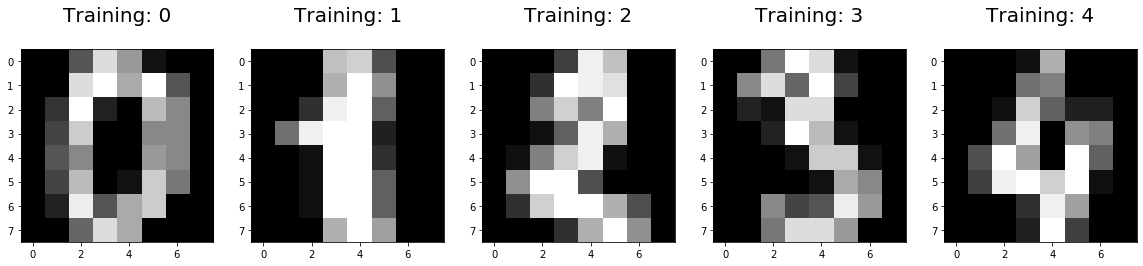#### Split into training and test¶¶

In :
from sklearn.model_selection import train_test_split
X_train, X_test, y_train, y_test = train_test_split(digits.data,
digits.target,
test_size=0.25,
random_state=0)

In :
X_train.shape, X_test.shape

Out:
((1347, 64), (450, 64))

#### Learning¶¶

Refer to the Logistic reg API ref for these parameters and the guide for equations, particularly how penalties are applied.

In :
from sklearn.linear_model import LogisticRegression
clf = LogisticRegression(fit_intercept=True,
multi_class='auto',
penalty='l2', #ridge regression
solver='saga',
max_iter=10000,
C=50)
clf

Out:
LogisticRegression(C=50, class_weight=None, dual=False, fit_intercept=True,
intercept_scaling=1, l1_ratio=None, max_iter=10000,
multi_class='auto', n_jobs=None, penalty='l2',
random_state=None, solver='saga', tol=0.0001, verbose=0,
warm_start=False)
In :
%%time
clf.fit(X_train, y_train)

CPU times: user 6.81 s, sys: 9.52 ms, total: 6.81 s
Wall time: 6.82 s

Out:
LogisticRegression(C=50, class_weight=None, dual=False, fit_intercept=True,
intercept_scaling=1, l1_ratio=None, max_iter=10000,
multi_class='auto', n_jobs=None, penalty='l2',
random_state=None, solver='saga', tol=0.0001, verbose=0,
warm_start=False)

Let us see what the classifier has learned

In :
clf.classes_

Out:
array([0, 1, 2, 3, 4, 5, 6, 7, 8, 9])
In :
clf.coef_.shape

Out:
(10, 64)
In :
clf.coef_.round(2) # prints weights for 8x8 image for class 0

Out:
array([ 0.  , -0.  , -0.04,  0.1 ,  0.06, -0.14, -0.16, -0.02, -0.  ,
-0.03, -0.04,  0.2 ,  0.09,  0.08, -0.05, -0.01, -0.  ,  0.06,
0.15, -0.03, -0.39,  0.25,  0.09, -0.  , -0.  ,  0.13,  0.16,
-0.18, -0.57,  0.02,  0.12, -0.  ,  0.  ,  0.16,  0.11, -0.16,
-0.41,  0.05,  0.08,  0.  , -0.  , -0.06,  0.27, -0.11, -0.2 ,
0.15,  0.04, -0.  , -0.  , -0.12,  0.08, -0.05,  0.2 ,  0.1 ,
-0.04, -0.01, -0.  , -0.01, -0.09,  0.21, -0.04, -0.06, -0.1 ,
-0.05])
In :
clf.intercept_ # for 10 classes - this is a One-vs-All classification

Out:
array([ 0.0010181 , -0.07236521,  0.00379207,  0.00459855,  0.04585855,
0.00014299, -0.00442972,  0.01179654,  0.04413398, -0.03454583])
In :
clf.n_iter_ # num of iterations before tolerance was reached

Out:
1876

#### Viewing coefficients as an image¶¶

Since there is a coefficient for each pixel in the 8x8 image, we can view them as an image itself. The code below is similar to the original viz code, but runs on coeff.

In :
coef = clf.coef_.copy()
plt.imshow(coef.reshape(8,8).round(2));  # proof of concept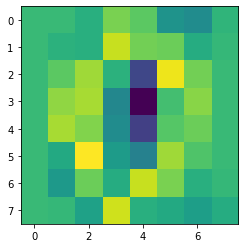In :
coef = clf.coef_.copy()
scale = np.abs(coef).max()
plt.figure(figsize=(10,5))

for i in range(10): # 0-9
coef_plot = plt.subplot(2, 5, i + 1) # 2x5 plot

coef_plot.imshow(coef[i].reshape(8,8),
cmap=plt.cm.RdBu,
vmin=-scale, vmax=scale,
interpolation='bilinear')

coef_plot.set_xticks(()); coef_plot.set_yticks(()) # remove ticks
coef_plot.set_xlabel(f'Class {i}')

plt.suptitle('Coefficients for various classes');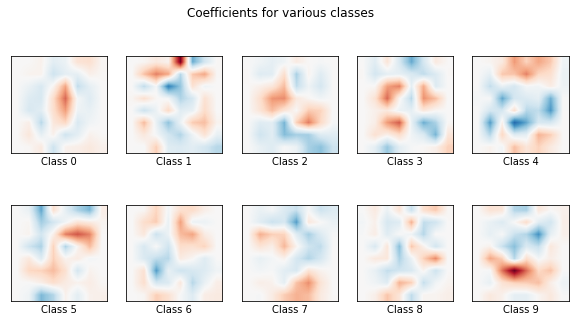#### Prediction and scoring¶¶

Now predict on unknown dataset and compare with ground truth

In :
print(clf.predict(X_test[0:9]))
print(y_test[0:9])

[2 8 2 6 6 7 1 9 8]
[2 8 2 6 6 7 1 9 8]


Score against training and test data

In :
clf.score(X_train, y_train) # training score

Out:
1.0
In :
score = clf.score(X_test, y_test) # test score
score

Out:
0.9555555555555556

Test score: 0.9555

##### Confusion matrix¶¶
In :
from sklearn import metrics

In :
predictions = clf.predict(X_test)

cm = metrics.confusion_matrix(y_true=y_test,
y_pred = predictions,
labels = clf.classes_)
cm

Out:
array([[37,  0,  0,  0,  0,  0,  0,  0,  0,  0],
[ 0, 40,  0,  0,  0,  0,  0,  0,  2,  1],
[ 0,  0, 42,  2,  0,  0,  0,  0,  0,  0],
[ 0,  0,  0, 43,  0,  0,  0,  0,  1,  1],
[ 0,  0,  0,  0, 37,  0,  0,  1,  0,  0],
[ 0,  0,  0,  0,  0, 46,  0,  0,  0,  2],
[ 0,  1,  0,  0,  0,  0, 51,  0,  0,  0],
[ 0,  0,  0,  1,  1,  0,  0, 46,  0,  0],
[ 0,  3,  1,  0,  0,  0,  0,  0, 43,  1],
[ 0,  0,  0,  0,  0,  1,  0,  0,  1, 45]])

Visualize confusion matrix as a heatmap

In :
import seaborn as sns

plt.figure(figsize=(10,10))
sns.heatmap(cm, annot=True,
linewidths=.5, square = True, cmap = 'Blues_r');

plt.ylabel('Actual label')
plt.xlabel('Predicted label')
all_sample_title = 'Accuracy Score: {0}'.format(score)
plt.title(all_sample_title);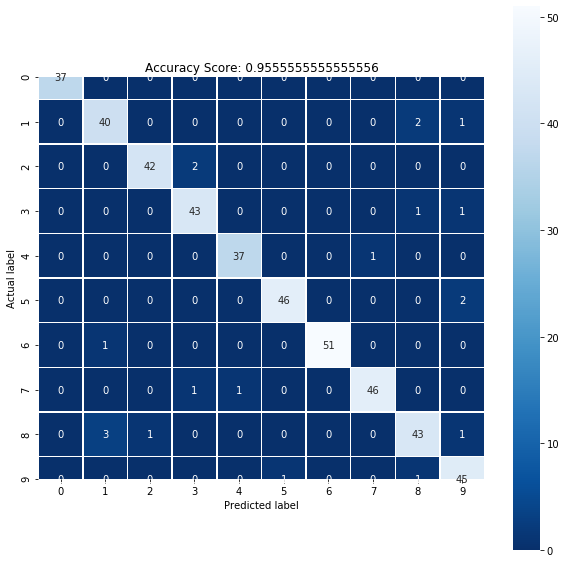##### Inspecting misclassified images¶¶

We compare predictions with labels to find which images are wrongly classified, then display them.

In :
index = 0
misclassified_images = []
for label, predict in zip(y_test, predictions):
if label != predict:
misclassified_images.append(index)
index +=1

In :
print(misclassified_images)

[56, 94, 118, 124, 130, 169, 181, 196, 213, 251, 315, 325, 331, 335, 378, 398, 425, 429, 430, 440]

In :
plt.figure(figsize=(10,10))
plt.suptitle('Misclassifications');

p = plt.subplot(4,5, plot_index+1) # 4x5 plot

interpolation='bilinear')
p.set_xticks(()); p.set_yticks(()) # remove ticks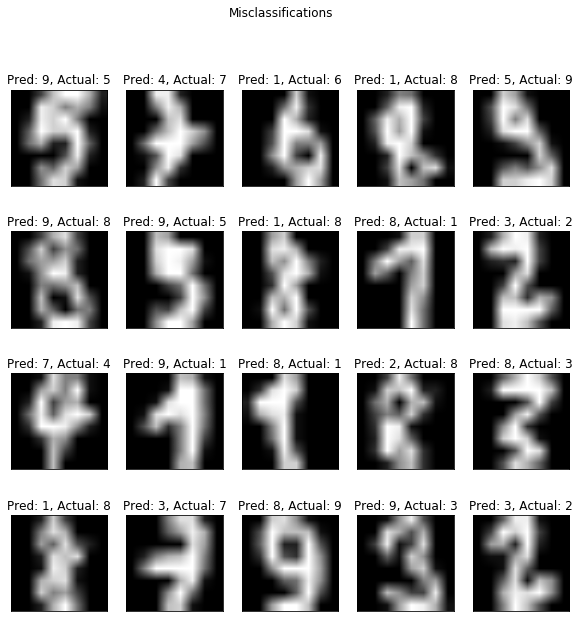### Predicting on full MNIST database¶¶

In the previous section, we worked with as tiny subset. In this section, we will download and play with the full MNIST dataset. Downloading for the first time from open ml db takes me about half a minute. Since this dataset is cached locally, subsequent runs should not take as much.

In :
%%time
from sklearn.datasets import fetch_openml
mnist = fetch_openml(data_id=554) # https://www.openml.org/d/554

CPU times: user 15.3 s, sys: 348 ms, total: 15.6 s
Wall time: 15.6 s

In :
type(mnist)

Out:
sklearn.utils.Bunch
In :
type(mnist.data), type(mnist.categories), type(mnist.feature_names), type(mnist.target)

Out:
(numpy.ndarray, dict, list, numpy.ndarray)
In :
mnist.data.shape, mnist.target.shape

Out:
((70000, 784), (70000,))

There are 70,000 images, each of dimension 28x28 pixels.

#### Preview some images¶¶

In :
mnist.target

Out:
'5'
In :
plt.figure(figsize=(20,4))
for index, (image, label) in enumerate(zip(mnist.data[0:5],
mnist.target[0:5])):
plt.subplot(1, 5, index + 1)
plt.imshow(np.reshape(image, (28,28)), cmap=plt.cm.gray)
plt.title('Training: ' + label, fontsize = 20);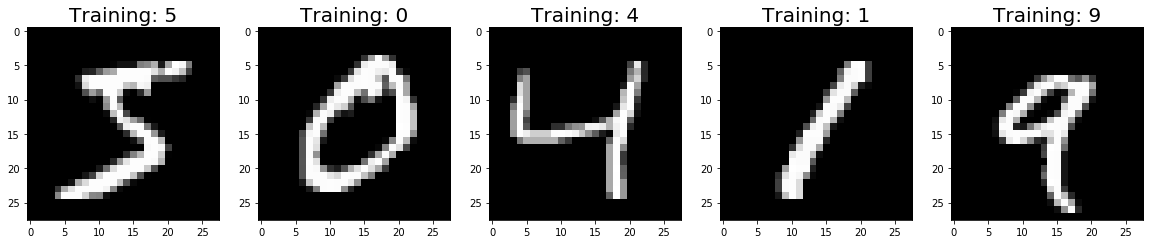#### Split into training and test¶¶

In :
mnist.target.astype('int')

Out:
array([5, 0, 4, ..., 4, 5, 6])
In :
from sklearn.model_selection import train_test_split
X2_train, X2_test, y2_train, y2_test = train_test_split(mnist.data,
mnist.target.astype('int'), #targets str to int convert
test_size=1/7.0,
random_state=0)

In :
X2_train.shape, X2_test.shape

Out:
((60000, 784), (10000, 784))

Are the different classes evenly distributed? We can find this by plotting a histogram of the labels in both test and training datasets.

In :
plt.figure(figsize=(10,5))
plt.subplot(1,2,1)
plt.hist(y2_train);
plt.title('Frequency of different classes - Training data');

plt.subplot(1,2,2)
plt.hist(y2_test);
plt.title('Frequency of different classes - Test data');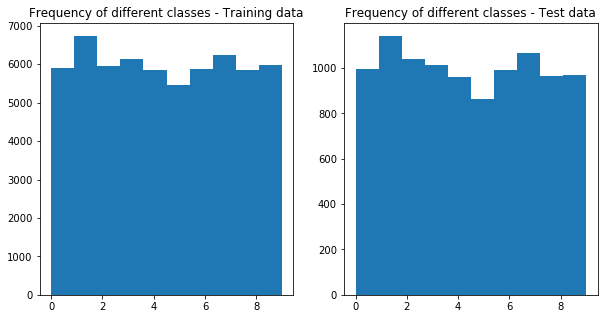#### Learning¶¶

In :
from sklearn.linear_model import LogisticRegression
clf2 = LogisticRegression(fit_intercept=True,
multi_class='auto',
penalty='l1', #lasso regression
solver='saga',
max_iter=1000,
C=50,
verbose=2, # output progress
n_jobs=5, # parallelize over 5 processes
tol=0.01
)
clf2

Out:
LogisticRegression(C=50, class_weight=None, dual=False, fit_intercept=True,
intercept_scaling=1, l1_ratio=None, max_iter=1000,
multi_class='auto', n_jobs=5, penalty='l1',
random_state=None, solver='saga', tol=0.01, verbose=2,
warm_start=False)

Since there are 10 classes and 12 available cores, we will try to run the learning step in 5 jobs. Earlier, when I did not parallelize, the job did not finish within 1 hour, when I had to put the machine to sleep for a meeting.

In :
%%time
clf2.fit(X2_train, y2_train)

[Parallel(n_jobs=5)]: Using backend ThreadingBackend with 5 concurrent workers.

convergence after 47 epochs took 143 seconds
CPU times: user 9min 30s, sys: 469 ms, total: 9min 30s
Wall time: 2min 22s

[Parallel(n_jobs=5)]: Done   1 out of   1 | elapsed:  2.4min finished

Out:
LogisticRegression(C=50, class_weight=None, dual=False, fit_intercept=True,
intercept_scaling=1, l1_ratio=None, max_iter=1000,
multi_class='auto', n_jobs=5, penalty='l1',
random_state=None, solver='saga', tol=0.01, verbose=2,
warm_start=False)

Note: Since the verbosity is set >0, the messages were printed, but they got printed on the terminal, not in the notebook.

Let us see what the classifier has learned

In :
clf2.classes_

Out:
array([0, 1, 2, 3, 4, 5, 6, 7, 8, 9])
In :
clf2.coef_.shape

Out:
(10, 784)

Get the coefficients for a single class, 1 in this case:

In :
clf2.coef_.round(3) # prints weights for 8x8 image for class 0

Out:
array([ 0.   ,  0.   ,  0.   ,  0.   ,  0.   ,  0.   ,  0.   ,  0.   ,
0.   ,  0.   ,  0.   ,  0.   ,  0.   ,  0.   ,  0.   ,  0.   ,
0.   ,  0.   ,  0.   ,  0.   ,  0.   ,  0.   ,  0.   ,  0.   ,
0.   ,  0.   ,  0.   ,  0.   ,  0.   ,  0.   ,  0.   ,  0.   ,
0.   ,  0.   ,  0.   , -0.   , -0.   , -0.   , -0.   , -0.   ,
-0.   , -0.   ,  0.   ,  0.   , -0.   , -0.   , -0.   , -0.   ,
-0.   ,  0.   ,  0.   ,  0.   ,  0.   ,  0.   ,  0.   ,  0.   ,
0.   ,  0.   ,  0.   ,  0.   ,  0.   , -0.   , -0.   , -0.   ,
-0.001, -0.001, -0.001,  0.   ,  0.002,  0.004,  0.001,  0.002,
0.002,  0.001, -0.   , -0.   , -0.   , -0.   , -0.   , -0.   ,
-0.   ,  0.   ,  0.   ,  0.   ,  0.   ,  0.   ,  0.   ,  0.   ,
-0.   , -0.   , -0.   , -0.001, -0.001, -0.002, -0.002, -0.003,
0.001,  0.002, -0.001,  0.001,  0.002,  0.   , -0.002,  0.   ,
-0.001, -0.001, -0.001, -0.001, -0.001, -0.   , -0.   ,  0.   ,
0.   ,  0.   ,  0.   , -0.   ,  0.   ,  0.001,  0.   , -0.   ,
-0.   , -0.   ,  0.   , -0.   ,  0.   ,  0.001,  0.   ,  0.   ,
-0.   ,  0.001, -0.001,  0.001,  0.   , -0.   , -0.001, -0.001,
-0.003, -0.002, -0.   , -0.   ,  0.   ,  0.   ,  0.   ,  0.   ,
0.   ,  0.003,  0.001, -0.002, -0.003, -0.002, -0.003, -0.003,
-0.002,  0.   , -0.001,  0.001, -0.001, -0.001,  0.   , -0.001,
0.   ,  0.   ,  0.002,  0.002, -0.001, -0.002, -0.   , -0.   ,
0.   ,  0.   , -0.   , -0.   ,  0.   ,  0.001,  0.   , -0.003,
-0.002, -0.001,  0.   ,  0.   , -0.002, -0.002, -0.001, -0.002,
-0.001, -0.003,  0.   , -0.001, -0.   , -0.   , -0.   ,  0.   ,
-0.001, -0.002, -0.   , -0.   ,  0.   , -0.   , -0.   , -0.   ,
0.   , -0.   , -0.002, -0.001, -0.003,  0.   , -0.   , -0.002,
0.001, -0.002,  0.001, -0.003,  0.   , -0.001, -0.002, -0.   ,
0.001,  0.   , -0.002, -0.001, -0.003, -0.002, -0.   , -0.   ,
0.   , -0.   , -0.   , -0.   ,  0.001, -0.001, -0.002,  0.001,
-0.002, -0.003, -0.001, -0.002, -0.   ,  0.001, -0.001,  0.   ,
-0.003,  0.001, -0.001,  0.001, -0.002, -0.001, -0.001, -0.003,
-0.004, -0.002, -0.   , -0.   , -0.   , -0.   , -0.   , -0.   ,
-0.   , -0.002, -0.001,  0.001,  0.   , -0.002, -0.001, -0.001,
-0.001, -0.   , -0.   ,  0.003,  0.001, -0.001,  0.   , -0.002,
-0.002, -0.001, -0.001, -0.003, -0.002, -0.002, -0.   , -0.   ,
-0.   , -0.   , -0.   , -0.001, -0.001, -0.003, -0.002, -0.001,
0.   , -0.001, -0.001,  0.001, -0.   ,  0.002,  0.003,  0.003,
0.002,  0.001, -0.001, -0.   , -0.002,  0.   , -0.001, -0.001,
-0.002, -0.001, -0.   ,  0.   ,  0.   ,  0.   , -0.   , -0.   ,
-0.001, -0.002, -0.004, -0.003, -0.002, -0.001, -0.001, -0.001,
-0.001,  0.001,  0.003,  0.003,  0.   , -0.001,  0.   , -0.001,
-0.   , -0.002, -0.001, -0.001, -0.001, -0.   , -0.   ,  0.   ,
0.   , -0.   , -0.   , -0.   ,  0.   , -0.001, -0.001, -0.001,
-0.001,  0.   , -0.001, -0.002, -0.   ,  0.002,  0.005,  0.003,
0.   , -0.   , -0.001, -0.001, -0.001, -0.   , -0.   , -0.   ,
-0.001, -0.   , -0.   ,  0.   ,  0.   , -0.   , -0.   ,  0.   ,
0.001,  0.   ,  0.   , -0.001,  0.   ,  0.001, -0.004, -0.002,
0.   ,  0.001,  0.002,  0.002,  0.001,  0.003, -0.003, -0.002,
0.   ,  0.   , -0.001, -0.001, -0.001, -0.   , -0.   , -0.   ,
0.   ,  0.   ,  0.   ,  0.   ,  0.001,  0.   , -0.001, -0.001,
0.001, -0.001, -0.003, -0.002, -0.   ,  0.001,  0.004,  0.002,
0.001, -0.   , -0.003, -0.003, -0.   , -0.001, -0.001, -0.001,
-0.   , -0.   , -0.   , -0.   ,  0.   ,  0.   ,  0.   ,  0.   ,
-0.   , -0.001, -0.001, -0.   ,  0.001, -0.002, -0.003, -0.   ,
0.001,  0.002,  0.004, -0.001,  0.003, -0.001, -0.002, -0.005,
-0.002, -0.001, -0.001, -0.001, -0.   , -0.   , -0.   , -0.   ,
0.   ,  0.   ,  0.   , -0.   , -0.   , -0.001, -0.001, -0.001,
-0.001,  0.001, -0.   , -0.   , -0.001,  0.001,  0.003, -0.002,
0.001, -0.005, -0.003, -0.003, -0.001, -0.   , -0.001, -0.001,
0.001, -0.001, -0.   , -0.   ,  0.   ,  0.   ,  0.   , -0.   ,
-0.   , -0.001, -0.002, -0.002, -0.001, -0.001,  0.   , -0.001,
0.001,  0.003,  0.002,  0.001, -0.001, -0.005, -0.001, -0.   ,
-0.   ,  0.   ,  0.   , -0.   , -0.   , -0.001,  0.   , -0.   ,
-0.   ,  0.   , -0.   , -0.   , -0.   , -0.003, -0.005, -0.003,
0.   ,  0.   , -0.002, -0.001,  0.   , -0.   ,  0.002, -0.001,
-0.003,  0.   ,  0.002,  0.   ,  0.002,  0.   , -0.001, -0.   ,
0.001,  0.002,  0.001,  0.   ,  0.   ,  0.   , -0.   , -0.   ,
-0.001, -0.005, -0.002,  0.001, -0.001,  0.001, -0.001, -0.001,
-0.   ,  0.002, -0.002, -0.001,  0.002, -0.001, -0.001,  0.001,
0.001, -0.001, -0.001,  0.   ,  0.002,  0.001,  0.001,  0.   ,
0.   , -0.   , -0.   , -0.   , -0.   , -0.005, -0.   ,  0.   ,
0.   ,  0.002,  0.003,  0.002,  0.001, -0.001,  0.001, -0.   ,
0.001,  0.002,  0.003,  0.001,  0.   , -0.001, -0.   ,  0.001,
0.001,  0.001,  0.001,  0.   ,  0.   ,  0.   , -0.   ,  0.001,
0.002,  0.003,  0.003,  0.002,  0.002, -0.001,  0.001, -0.001,
-0.   ,  0.001,  0.002,  0.001,  0.001,  0.001,  0.003,  0.001,
0.003,  0.003, -0.001,  0.   ,  0.003,  0.001,  0.   ,  0.   ,
0.   ,  0.   , -0.   ,  0.   ,  0.001,  0.007,  0.003,  0.   ,
-0.   ,  0.001, -0.002, -0.001, -0.002, -0.004, -0.001, -0.   ,
-0.   ,  0.   ,  0.002,  0.001,  0.003,  0.002, -0.   , -0.   ,
0.001,  0.001,  0.   ,  0.   ,  0.   ,  0.   , -0.   , -0.001,
-0.002,  0.002,  0.001,  0.001,  0.   ,  0.001,  0.   ,  0.   ,
0.001,  0.001, -0.001,  0.002,  0.001,  0.002, -0.   ,  0.   ,
-0.001, -0.001, -0.001, -0.001, -0.   , -0.   ,  0.   ,  0.   ,
0.   ,  0.   ,  0.   , -0.001, -0.001, -0.001, -0.001, -0.   ,
-0.001,  0.001, -0.001, -0.001, -0.001, -0.001, -0.001, -0.   ,
-0.004,  0.001,  0.001,  0.   , -0.001, -0.001, -0.001, -0.   ,
-0.   ,  0.   ,  0.   ,  0.   ,  0.   ,  0.   ,  0.   , -0.   ,
-0.   , -0.001, -0.001, -0.002, -0.001, -0.003, -0.006, -0.004,
-0.001, -0.002, -0.002, -0.003, -0.004, -0.003, -0.002, -0.002,
-0.001, -0.   , -0.   , -0.   , -0.   ,  0.   ,  0.   ,  0.   ,
0.   ,  0.   ,  0.   , -0.   , -0.   , -0.   , -0.   , -0.   ,
-0.   , -0.   , -0.001, -0.001, -0.002, -0.002, -0.001, -0.001,
-0.   , -0.   , -0.001, -0.001, -0.   , -0.   , -0.   , -0.   ,
0.   ,  0.   ,  0.   ,  0.   ,  0.   ,  0.   ,  0.   ,  0.   ,
0.   ,  0.   , -0.   , -0.   , -0.   , -0.   , -0.   , -0.   ,
-0.   , -0.   , -0.   , -0.   , -0.   , -0.   , -0.   , -0.   ,
-0.   ,  0.   ,  0.   ,  0.   ,  0.   ,  0.   ,  0.   ,  0.   ])
convergence after 591 epochs took 1805 seconds

In :
clf2.intercept_ # for 10 classes - this is a One-vs-All classification

Out:
array([-1.11398188e-04,  1.38709472e-04,  1.16909054e-04, -2.37842193e-04,
6.62466316e-05,  8.48133979e-04, -4.22181499e-05,  2.66499796e-04,
-8.62715013e-04, -1.82325388e-04])
In :
clf2.n_iter_ # num of iterations before tolerance was reached

Out:
47
##### Visualize coefficients as an image¶¶
In :
coef = clf2.coef_.copy()
scale = np.abs(coef).max()
plt.figure(figsize=(13,7))

for i in range(10): # 0-9
coef_plot = plt.subplot(2, 5, i + 1) # 2x5 plot

coef_plot.imshow(coef[i].reshape(28,28),
cmap=plt.cm.RdBu,
vmin=-scale, vmax=scale,
interpolation='bilinear')

coef_plot.set_xticks(()); coef_plot.set_yticks(()) # remove ticks
coef_plot.set_xlabel(f'Class {i}')

plt.suptitle('Coefficients for various classes');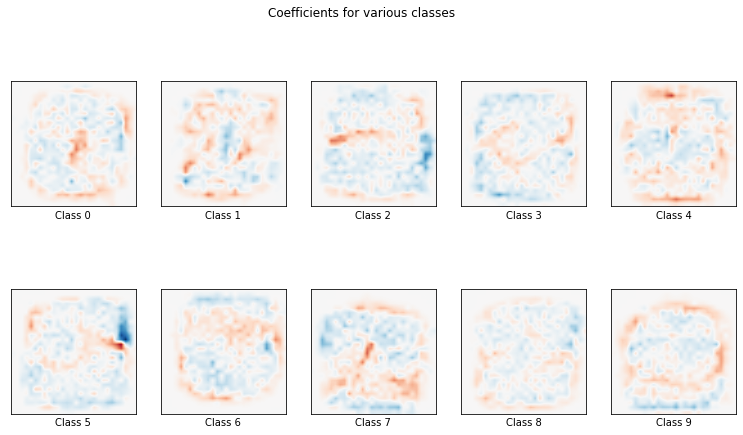#### Prediction and scoring¶¶

Now predict on unknown dataset and compare with ground truth

In :
print(clf2.predict(X2_test[0:9]))
print(y2_test[0:9])

[0 4 1 2 4 7 7 1 1]
[0 4 1 2 7 9 7 1 1]


Score against training and test data

In :
clf2.score(X2_train, y2_train) # training score

Out:
0.9374333333333333
In :
score2 = clf2.score(X2_test, y2_test) # test score
score2

Out:
0.9191

Test Score: 0.9191 or 91%

#### Confusion matrix¶¶

In :
from sklearn import metrics

In :
predictions2 = clf2.predict(X2_test)

cm = metrics.confusion_matrix(y_true=y2_test,
y_pred = predictions2,
labels = clf2.classes_)
cm

Out:
array([[ 967,    0,    1,    2,    1,    9,    9,    0,    7,    0],
[   0, 1114,    5,    3,    1,    5,    0,    4,    7,    2],
[   3,   13,  931,   18,   11,    1,   15,   10,   34,    4],
[   1,    5,   33,  894,    0,   26,    2,   12,   27,   13],
[   1,    2,    5,    1,  897,    1,   11,    9,    7,   28],
[  10,    2,    6,   30,    9,  747,   16,    6,   30,    7],
[   7,    3,    6,    0,   11,   18,  938,    1,    5,    0],
[   2,    5,   13,    2,   11,    2,    1,  982,    4,   42],
[   4,   18,    8,   18,    6,   25,    9,    2,  861,   12],
[   3,    5,    6,   10,   35,    7,    2,   32,    9,  860]])
In :
import seaborn as sns

plt.figure(figsize=(12,12))
sns.heatmap(cm, annot=True,
linewidths=.5, square = True, cmap = 'Blues_r', fmt='0.4g');

plt.ylabel('Actual label')
plt.xlabel('Predicted label')
all_sample_title = 'Accuracy Score: {0}'.format(score2)
plt.title(all_sample_title);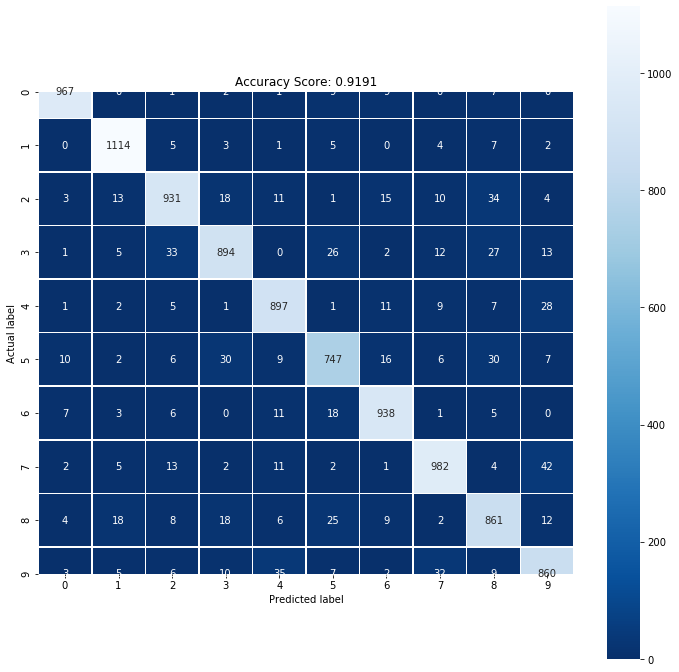convergence after 5470 epochs took 10856 seconds


### Conclusion¶¶

This notebook shows performing multi-class classification using logistic regression using one-vs-all technique. When run on MNIST DB, the best accuracy is still just 91%. There is still scope for improvement.